# Linear functional

(diff) ← Older revision | Latest revision (diff) | Newer revision → (diff)

linear form, on a vector spaceover a fieldA mapping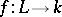such that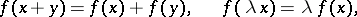for all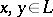,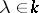. The concept of a linear functional, as an important special case of the concept of a linear operator, is one of the main concepts in linear algebra and plays a significant role in analysis.

On the set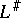of linear functionals onthe operations of addition and multiplication by a scalar are defined according to the formulas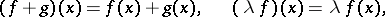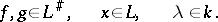They specify ina vector space structure over.

The kernel of a linear functional is the subspace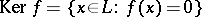. If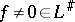(that is,), then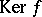is a hyperplane in. Linear functionals with the same kernel are proportional.

If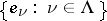is a basis of, then for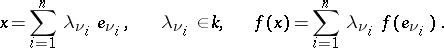The correspondenceis an isomorphism ofonto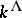. Corollary:is isomorphic toif and only if it is finite dimensional. On transition to a new basis inthe elements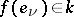are transformed by the same formulas as the basis vectors.

The operator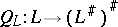defined by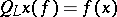is injective. It is an isomorphism if and only ifis finite dimensional. This isomorphism, in contrast to the isomorphism betweenand, is natural, i.e. functorial (cf. Functorial morphism).

A linear functional on a locally convex space, in particular on a normed space, is an important object of study in functional analysis. Every continuous (as a mapping on topological spaces) linear functionalon a locally convex spaceis bounded (cf. Bounded operator), that is,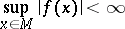for all bounded. Ifis a normed space, the converse is also true; both properties are then equivalent to the finiteness of the numberThe continuous linear functionals on a locally convex spaceform a subspace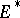of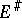, which is said to be the dual of. Inone considers different topologies, including the weak and strong topologies, which correspond, respectively, to pointwise and uniform convergence on bounded sets. Ifis a normed space, thenis a Banach space with respect to the norm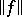and the corresponding topology coincides with the strong topology. The unit ball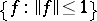, considered in the weak topology, is compact.

The Hahn–Banach theorem has important applications in analysis; one formulation of it is as follows: If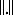is a pre-norm on a vector spaceand ifis a linear functional defined on a subspaceofsuch that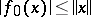for all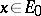, then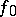can be extended to the whole of, preserving linearity and the given bound. Corollary: Any continuous linear functional defined on a subspaceof a locally convex spacecan be extended to a continuous linear functional on, and ifis a normed space, then the norm is preserved. Hence, for every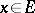,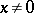, there is an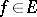with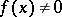.

Letbe a normed space and suppose that, and then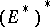, are taken with the corresponding norms. Then the operator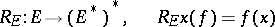is an isometric imbedding. If under this imbeddingcoincides with, then, which is necessarily complete, is said to be reflexive (cf. Reflexive space). For example,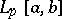and,, are reflexive if and only if. There is a similar concept of reflexivity for general locally convex spaces.

For many locally convex spaces, all linear functionals have been described. For example, the adjoint of a Hilbert spaceis. The adjoint ofis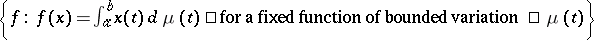.

How to Cite This Entry:
Linear functional. Encyclopedia of Mathematics. URL: http://encyclopediaofmath.org/index.php?title=Linear_functional&oldid=14314
This article was adapted from an original article by A.Ya. Khelemskii (originator), which appeared in Encyclopedia of Mathematics - ISBN 1402006098. See original article